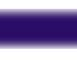in Ecology and Evolution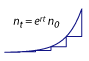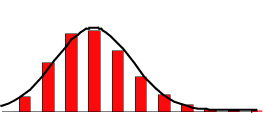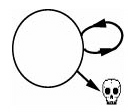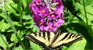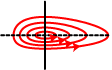Samples Additional material Welcome The aim of our book is to teach why mathematics is so useful in biology, how to read and interpret equations, and how to construct and analyze new models. The book is fairly comprehensive, providing the background needed to analyze a wide variety of models. Our focus is on developing ecological and evolutionary models that describe how biological systems change over time. That said, most of the techniques presented in the book can be readily applied to model other phenomena, in biology as well as in other disciplines. The first six chapters and Primer 1 introduce the art of constructing and analyzing a model. This material assumes that you have had elementary algebra and calculus, although we review techniques as needed. The next section (Chapters 7-12) covers more advanced models that describe multiple classes of individuals and interactions among them. For this material, an understanding of how to handle matrices and vectors is necessary. Because most biologists have not had a course on matrices, Primer 2 introduces important concepts and methods of matrix algebra. We end the book with a section (Chapters 13-15) on probabilistic modeling in biology, starting with a Primer 3 introducing probability theory. About the Authors About the Cover Art Ordering (paid link) Order within Canada Order from Princeton University Press

 biomath www